# Статьи журнала - Пространство, время и фундаментальные взаимодействия

Все статьи: 278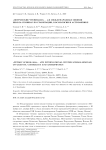"Петровские чтения-2018" - 4-я международная зимняя школа-семинар по гравитации, космологии и астрофизике

Сушков С.В., Хапаева А.В., Червон С.В., Бусиль Л.А.

Статья обзорная

В данной статье представлен обзор работы 4-ой Международной зимней школы-семинара по гравитации, космологии и астрофизике "Петровские чтения-2018" и мероприятий, приуроченных к 70-летию академика А.А. Старобинского.

Бесплатно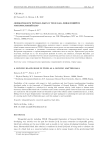A moving black hole in TEGR as a moving matter ball

Emtsova E.D., Petrov A.N.

Статья научная

Possibilities of the covariant with respect to both coordinate and local Lorentz transformations formalism developed earlier in the framework of Teleparallel Equivalent of General Relativity (TEGR) are studied. The formalism is applied to a solution for a moving with constant velocity (with respect to distant static observers) Schwarzschild black hole. Coordinate and Lorentz invariant global conserved mass and momentum are constructed. The acceptable results are obtained in spite of the solution under consideration has no, at least, Killing vectors of space displacements. Calculations are quite analogous to calculating the mass and momentum of a moving matter ball in Minkowski space, and this analogy is used essentially.

Бесплатно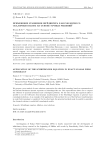Application of the Schrodinger equation in exact scalar field cosmology

Fomin I.V., Chervon S.V., Maharaj S.D.

Статья научная

We propose a new method of exact solutions construction for scalar field cosmology based on representation of the Einstein-Friedmann dynamic equations as Schr¨odinger-like one. This representation allows one to compare the solutions of quantum-mechanical and cosmological problems. On the other hand, this approach makes it possible to use the well-known form-invariant transformations of the Schr¨odinger equation to generate exact cosmological solutions. As an example of the application of this method, the use of the Darboux transformations in scalar field cosmology is considered. On the other hand, the presented methods make it possible to generalize the obtained solutions to multi-field cosmological models.

Бесплатно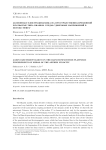Axion electrodynamics on the Bianchi spacetime platform: fingerprints of shear of the aether velocity

Shakirzyanov A.F., Balakin A.B.

Статья научная

In the framework of axionically extended Einstein-Maxwell-aether theory we study the structure of the electromagnetic field allowed by the anisotropic cosmological spacetime platforms associated with the Bianchi models. These models guarantee that the aether velocity possesses the shear, and we focus on its role in the evolution of the axion-photon systems. In this short note we discuss the extended master equations obtained under the assumption that the square of the shear tensor is included in the potential of the axion field.

Бесплатно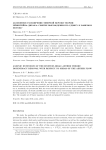Axionic extension of the Einstein-Dirac-aether theory: degeneracy removal with respect to shear of the aether flow

Efremova A.O., Balakin A.B.

Статья научная

We consider dynamics of the quartet of interacting cosmic substrata, which includes the dynamic aether, presented by the unit timelike vector field, the axionic dark matter, described by the pseudoscalar field, the spinor field associated with fermion particles, and the gravity field. The extended set of master equations is derived based on the idea that the potential of the axion field to be the function of seven arguments. The first one is, standardly, the pseudoscalar field; the second and third arguments are the fundamental spinor invariant and pseudoinvariant; the fourth and fifth ones are the aether-axion cross-invariants and cross-pseudoinvariants; the sixth argument is the expansion scalar, and the seventh one is the square of tensor of the shear of the aether flow. The complete set of Master equations is derived and prepared for analysis.

Бесплатно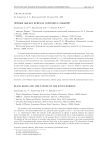Black holes and the nature of the event horizon

Andrusenko S.L., Krichevskiy D.P., Rudenko V.N.

Статья научная

The problem of the event horizon in relativistic gravity is discussed. Singular solutions in general relativity are well known. The Schwarschild metric of a spherical mass is singular at zero (𝑟 = 0) and at the event horizon (𝑟 = 𝑟𝑔). Both features reflect the existence of the phenomenon of collapse in general relativity for compact masses exceeding 3𝑀⊙. A material particle crossing the event horizon falls into a central singularity according to the classical theory of general relativity. In the quantum theory of gravity, there may be no central singularity. The physics of the event horizon is currently being refined. A promising technique is the study of gravitational waves (GW) accompanying the merger of binary black holes at the ringdown stage. GW observations of quasinormal modes of the newly formed super dense remnant will help clarify the physics of the event horizon.

Бесплатно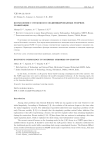Bouncing cosmology in modified theories of gravity

Mishra B., Agrawal A., Tripathy S.K.

Статья научная

In this study, we describe a 𝑓(𝑅) gravity theory-based bouncing cosmological model of the universe. The flat FLRW space-time were used to determine the model’s dynamical behaviour. At the bouncing epoch, the geometrical parameters exhibit singularity behaviour. The parameters of the scale factor have a significant impact on the bouncing behaviour.

Бесплатно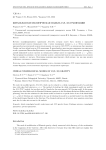Chiral cosmological model of F(R,R) gravity

Chervon S.V., Fomin I.V., Chaadaeva T.I.

Статья научная

We study the modified gravity 𝑓(𝑅,□𝑅), which can be reduced to a chiral cosmological model of a particular type with scalar field observers 𝜒, 𝜑, 𝜙. The method of studying the chiral cosmological model was used for the 𝑓(𝑅, (∇𝑅)2) models, but was first used by Naruko for the more general 𝑓(𝑅) Gracing model. In this paper, equations for chiral fields and the Einstein-Friedmann equations in the Friedmann-Robertson-Walker metric are presented. Note that the solutions for this chosen model are based on a special choice of one of the chiral fields: = - √ 3/2 ln 2. Solutions are considered in the case of reducing the model to a one-field model: two chiral fields of the model depend linearly on the third one. The obtained solutions are of interest, since quantum corrections must also be taken into account in the analysis.

Бесплатно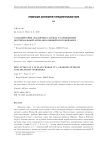Cамодействие скалярного заряда в заряженной экстремальной анти-дилатонной кротовой норе

Аслан О., Попов А.А.

Статья научная

Вычислена сила самодействия скалярного заряда в пространство-времени экстремальной заряженной анти-дилатонной кротовой норы. Предполагается, что скалярный заряд является источником безмассо- вого скалярного поля, минимально связанного с кривизной пространства-времени.

Бесплатно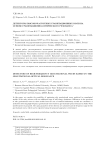Detectors of high-frequency gravitational waves based on the gravitational-optical resonance

Morozov A.N., Golyak I.S., Fomin I.V., Chervon S.V.

Статья научная

In this paper, we consider the possibility of registering relic gravitational waves predicted in cosmological models of the early universe with an additional stage of stiff energy dominance. Differences in the spectrum of relic gravitational waves in these models from the case of standard inflationary models are considered. A method for detecting high-frequency gravitational waves based on gravitational-optical resonance in Fabry-Perot interferometers is proposed. The main parameters of the detector are calculated and the frequency range of relic gravitational waves, which can be registered using the proposed approach, is determined.

Бесплатно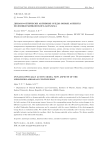Dynamo-optically active media: new aspects of the Minkowski-Abraham controversy

Alpin T.Y., Balakin A.B.

Статья научная

Based on the covariant variation formalism, two versions of the symmetric effective stress-energy tensor of the electromagnetic field in a dynamo-optically active relativistic media are reconstructed in the framework of the tetrad and aether paradigms, respectively. We show that the energy density scalars and pressure tensors coincide for both versions of the stress-energy tensors, however, the corresponding energy flux four-vectors happen to be different in general case. This mathematical fact adds new arguments into the 100-year-long discussion, which is called Minkowski-Abraham controversy and is connected with the correct definition of the electromagnetic energy flux in a continuous media. We consider three examples: first, the axionically active vacuum; second, the spatially isotropic moving dielectric medium; third, the dynamo-optically active medium. We discuss possible applications of the elaborated formalism.

Бесплатно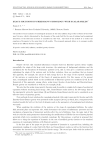Exact solutions in Friedmann cosmology with scalar fields

Fomin I.V.

Статья научная

The method of exact analysis of cosmological dynamics at the early inflation stage of the evolution of the Friedman Universe, which is determined by the dynamics of the scalar field for the case of minimal and nonminimalinteraction of the field and curvature is considered in this work. The basis of the method is to reduce theequations of dynamics to the same form for all models. The proposed approach allows us to compare modelsbased on the different theories of gravity.

Бесплатно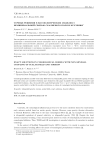Exact solutions in cosmological models with non-minimal coupling of scalar field and torsion

Dentsel E.S., Fomin I.V.

Статья научная

Cosmological inflation models with scalar field based on teleparallel gravity with non-minimal coupling of scalar field and torsion are considered. Solution type obtained in this paper is justified for any Hubble parameter and scalar field evolution. Also, the model with scalar field potenital (𝜑) = 𝑚2 2 𝜑𝑛, relating to chaotic inflation with massive scalar field, is verified by modern restrictions on values of cosmological perturbations parameters.

Бесплатно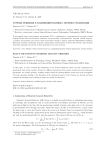Exact solutions in modified gravity theories

Ripathy S.K., Mishra B.

Статья научная

In this paper, we have reviewed the limitations in the General Relativity theory and the requirement for geometrical dark energy models. Actions for different geometrical dark energy models have been discussed. Specifically, two matter geometry coupling models within the Einstein-Hilbert action have been investigated. The basic equations leading to the discussion of the dynamical aspects of the Universe are derived and some examples of obtaining exact solutions in these two models are discussed.

Бесплатно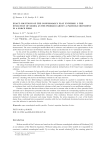Exact solutions of the conformally flat universe. I. The evolution of model as the problem about a particle movement in a force field

Baranov A.M., Saveljev E.V.

Статья научная

The problem reduction of an evolution modelling of the open Universe for conformally flat space-time metric in Fock’s form to an equivalent problem of a particle movement with an unit mass in a force field is demonstrated. The exact cosmological models filled with a substance and radiation in an approximation of the perfect fluid are found since the Friedman solution by means an introduction of set "mechanical" potentials.In the article the possibility of deriving from the Einstein equations exact cosmological solutions for the open Universe by reduction to the equivalent problem of a mass particle motion in the force field is considered. The cosmological model is filled by substance in an approximation of the perfect fluid with nonzero pressure, generally speaking. The metric of 4D space-time is taken in the Fock form as the metric conformal to the Minkowski metric. This metric has the dependence on one variable. A square of the variable is product of advanced and retarded times.The using of mechanical interpretation of the gravitation equations leads to a possibility of consideration of various mechanics force fields with the subsequent physical interpretation of the found exact cosmological solutions.First of all a movement of a free particle with an unit mass (a mechanical force equals to zero) is considered,i.e. the particle moves on inertia. The fourth degree of discovered law of movement is a conformal factor of the cosmological metric which is conformally flat. This case corresponds to the exact cosmological solution without pressure, coinciding with known the Friedman solution for the open Universe.After that the force field leading to uniformly decelerated motion of a particle is considered. The force potential is taken in the form of linear function. The tangent of a slope angle of the function curve coincides with particle acceleration. Such research leads to the exact cosmological solution asymptotically describing both an incoherent dust, and the ultrarelativistic substance which may be interpreted as an equilibrium radiation.Further a square-law function without a linear term and a constant value is taken as a force potential. Such potential can be interpreted as potential of the free oscillator. The solution of corresponding equation of motion is written down in the form of a cosine function with some initial phase related to the ratio between parameters which define dust-like and ultrarelativistic substance. This conclusion becomes obvious after concidering asymptotic behaviour of pressure and energy density. Besides, the series expansion of a root of the fourth degree from a conformal factor asymptoticly coincides with the law of uniformly decelerated motion in previous case that indicates its particular character.

Бесплатно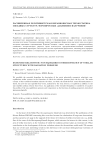Extended relativistic non-equilibrium thermostatics of stellar structures with radiation pressure

Balakin A.B., Tukbaev Z.Z.

Статья научная

We establish the extended formalism for description of the static spherically symmetric relativistic non-equilibrium stellar systems in the formation of which the radiation pressure plays the key role. The main concept of this extended formalism inherits the ideas, on which the Israel-Stewart causal thermodynamics is based, but now the unit spacelike four-vector, indicated by the term director, is exploited in addition to the unit timelike medium velocity four-vector. An application of the extended formalism is considered; we analyze the profiles of the non-equilibrium pressure and temperature as the functions of guiding parameters introduced phenomenologically.

Бесплатно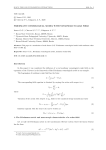Friedmann cosmological model with nonlinear scalar field

Ivanov G.G., Chervon S.V., Khapaeva A.V.

Статья научная

This paper is a translation of work Ivanov G.G "Friedmann cosmological model with nonlinear scalar field" of 1981 .

Бесплатно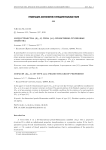H-spaces (H41,G) of type {41}: projective-group properties

Aminova A. V., Khakimov D. R.

Статья научная

In this paper we study five-dimensional ℎ-spaces (𝐻41, 𝑔) of type {41} . Necessary and sufficient conditions for (𝐻41, 𝑔) to be a space of constant curvature are found. The general solution of the Eisnhart equation in ℎ-space (𝐻41, 𝑔) of non-constant curvature is determined. We establish conditions for the existence of a non-homothetic projective motion in (𝐻41, 𝑔) and describe the structure of a non-homothetic projective Lie algebra in ℎ-space (𝐻41, 𝑔) of type {41}.

Бесплатно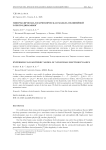Hyperbolic-logarithmic model of nonlinear electrodynamics

Groshev D.E., Spasov D.A.

Статья научная

In this paper we consider a new model of nonlinear electrodynamics - "Hyperbolic-logarithmic". This model contain a three parameters and describe by following Lagrangian: ℒ = -ℱ - 𝛽 𝑎𝑟𝑡ℎ(𝛽ℱ) - 2𝛽 [ln(1 + 𝛽ℱ) + ln(1-𝛽ℱ)], where ℱ = 1 4𝐹𝑖𝑘𝐹𝑖𝑘. We show, that in this model dual symmetry is broken. Also we proved that the electric field of a point-like charge becomes non-singular in this framework, static electric energy of this charge is finite. We calculate a theory parameters values guided by electron parameters and Abraham - Lorentz idea about a pure electromagnetic nature of electron mass. We find the canonical and symmetrical Belifante energy momentum tensors.

Бесплатно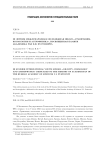III летняя международная молодежная школа «Гравитация, космология и астрофизика», посвященная памяти академика ран В.И. Пустовойта

Гладышев В.О., Фомин И.В.

Статья обзорная

В данной статье представлен обзор работы III летней международной молодежной школы «Гравитация, космология и астрофизика», посвященной памяти академика РАН В.И. Пустовойта.

Бесплатно

Журнал